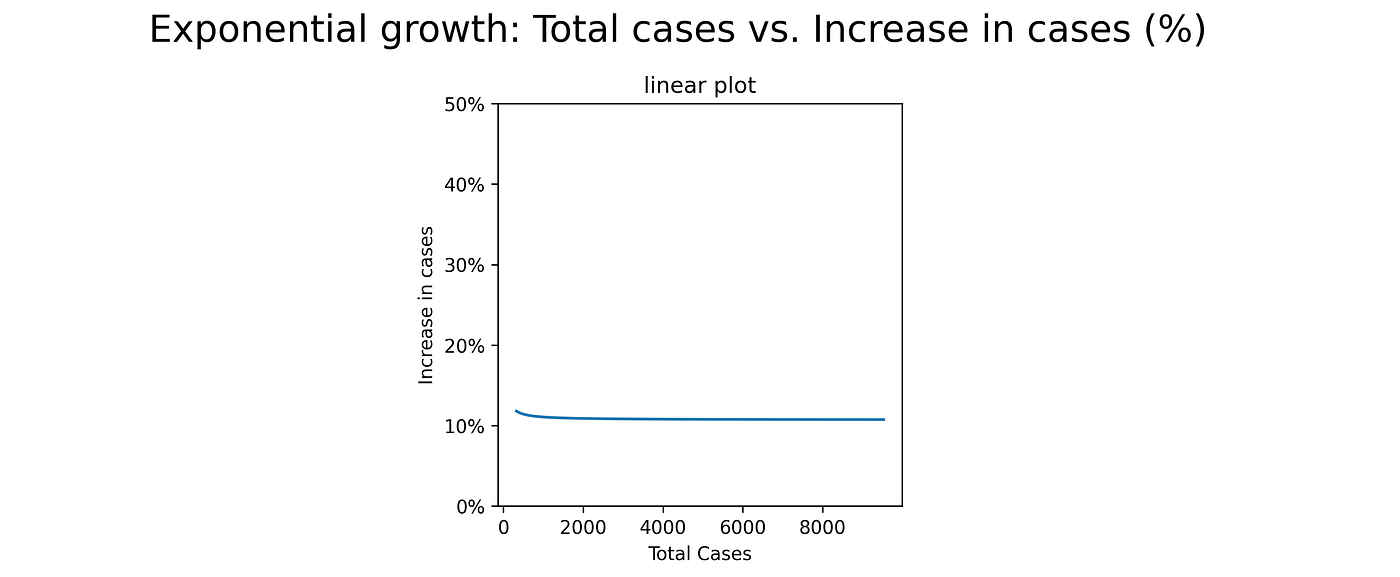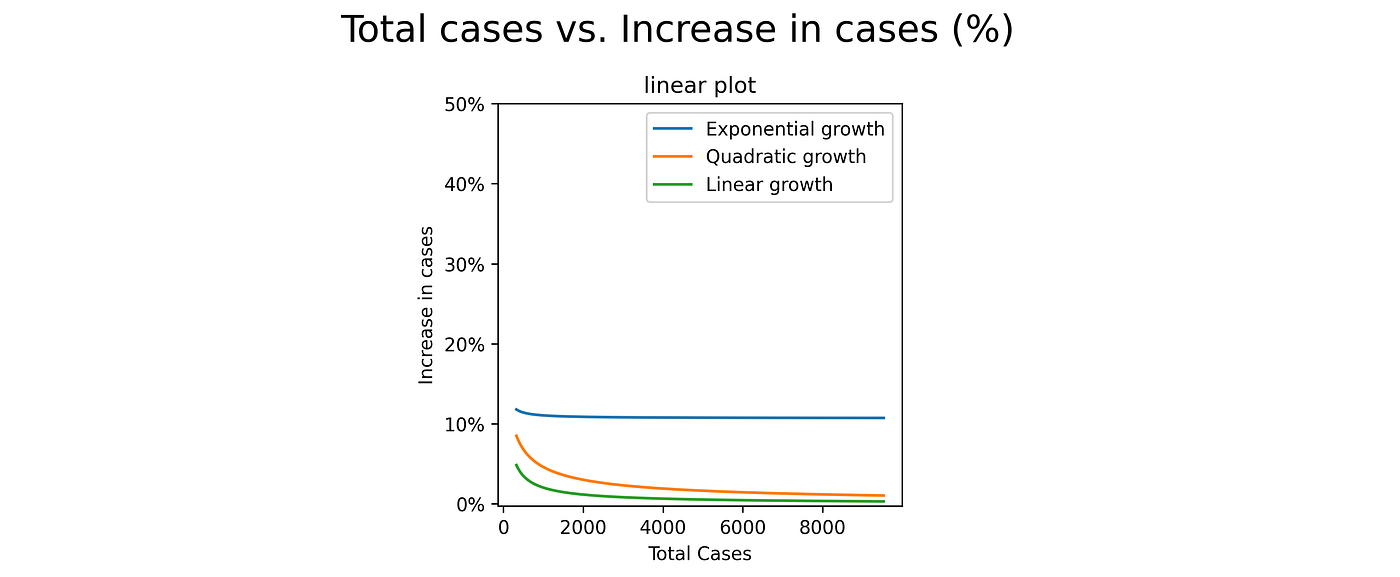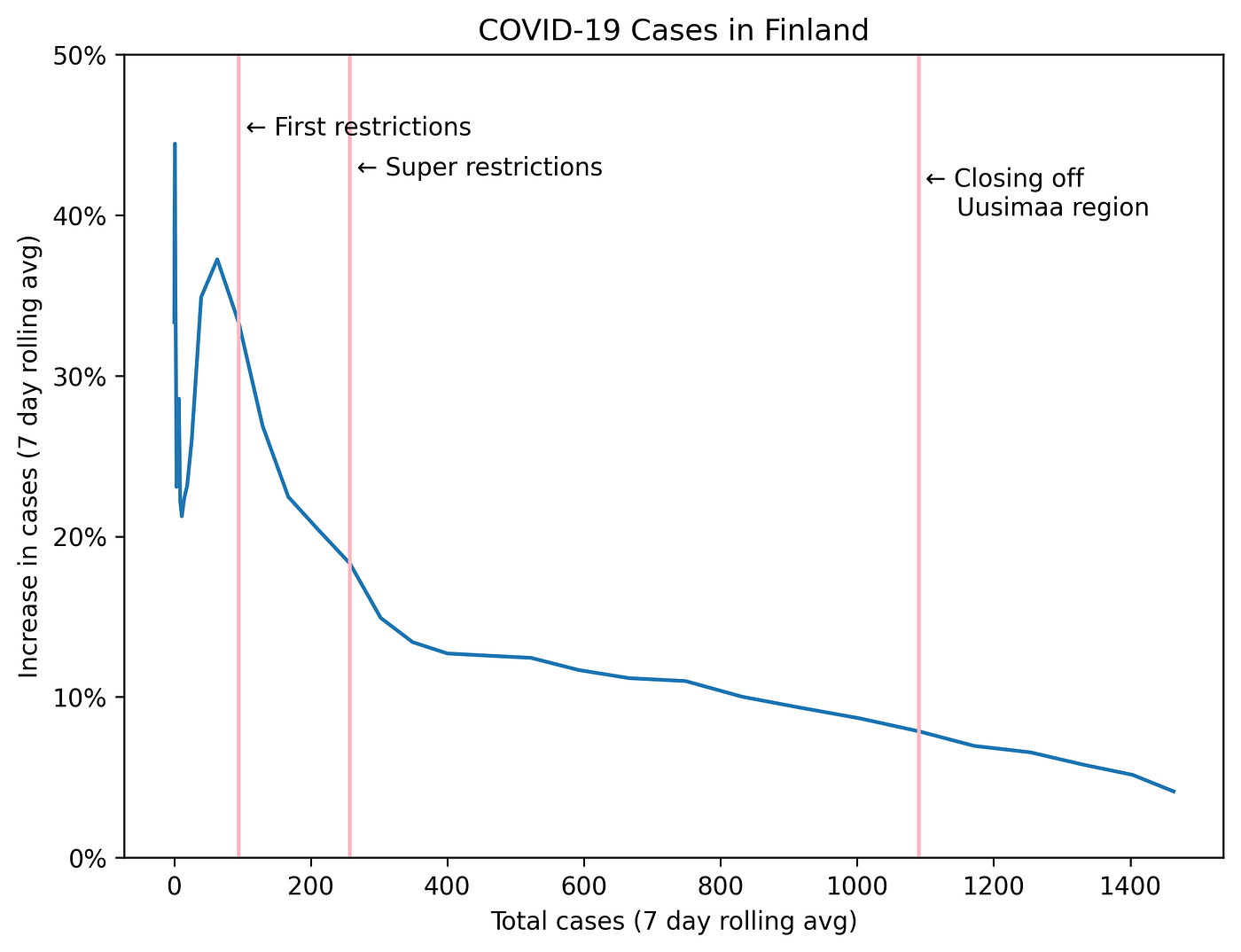# Types of Growth and How to Show Them

As the number of COVID-19 cases has increased, so has the number of dashboards, graphs and people who do them. While most of it has been more-of-the-same, some have managed to convey new insights. As the graphs and transformations get more complex, though, it is easy to lose sight of what we are actually looking at.

# Linear vs. Logarithmic Scales

On a linear scale, steps of fixed value (e.g. 10, 20, 30) are spaced the same distance apart. On a logarithmic scale, the equidistant steps are multiples: 10, 100, 1000 — in this case multiples of 10, but some other multiplier can be used as well.

In graphs, either y-axis, x-axis or both can have logarithmic scales. If it is just one of the axes, they can be called semi-logarithmic graphs or plots, and if both axes have logarithmic scales, log-log plots.

# Linear Growth

Linear growth might be the one we are most familiar with: If you save a fixed amount each month, linear growth if what happens in your savings account.

On a linear plot — both axes have linear scales — it is seen a straight line, with the slope corresponding to the term a in y = ax. Things get more confusing in the semi-log (linear x-axis, logarithmic y-axis) and log-log plots: You cannot estimate the slope easily from those plots, and not necessarily even see that the type of growth is linear.

# Quadratic growth and polynomial growth

Quadratic growth — of the form y(x) = x² — is familiar to us at least from physics: With constant acceleration, as in free fall (not taking air resistance into account), the distance increases quadratically.

The parabolic shape seen in the linear plot should be familiar to us: Kicking or throwing a ball happens in parabolic curve (well, what happens after, anyway).

Linear growth and quadratic growth are both special cases of polynomial growth: y(x)=ax + bx² + … + cxⁿ+C. The usefulness of log-log plots can be seen when estimating the highest exponent term, i.e. the degree of the polynomial. The slope of the curve corresponds to the degree of the polynomial.

# Exponential Growth

Now we are getting to the meat & potatoes of our pandemic times: Exponential growth. This is what happens when growth of an organism is not limited by environmental factors: For simple organisms like bacteria and cells that make up larger organisms, reproduction happens by division: one becomes two, two become four and so on. As long as, on average, the number of surviving cells after division is greater than one, exponential growth appears.

Here we see the usefulness of linear-logarithmic plots: They allow us to drop and exponential growth back to linear realm, and compare more easily exponential phenomena that in different stages or growing at different rates: These kinds of plots were and are most commonly used to show the progression of the COVID-19 epidemic.

The caveat is that, if we are not used to seeing linear-logarithmic plots, it can be hard to appreciate how drastic seemingly small differences are in absolute numbers: The main benefit of allowing us to compare numbers of different magnitude is also a drawback, if we are not used to seeing these kinds of plots.

# Fancy Graphs: Innovation in the Age of COVID-19

As the news on COVID-19 is taking over the media, the data journalism is also taking steps to shed new light on the phenomena.

Aatish Bhatia, in collaboration with minutephysics, has opted to turn the phenomena into linear realm by plotting New cases against Total confirmed cases (at the time). In more mathematical terms, you can say that the number of new cases is proportional to total existing cases at the time, or New cases ~ Existing cases.

They also opted to use a log-log plot: to compare countries that have not only vastly different number of total cases, but also increase/day. They are also showing the effect of time, animating the graph showing the curve up to and including each day.

Their rationale for the choices, as well as elaboration of caveats resulting from those, can be seen on a minutephysics video:

New York Times, on the other hand, took this approach a step further: Instead of showing New cases ~ Existing cases, they opted to plot Change in number of cases (%). Effectively, you are dividing the number of New cases by Existing cases: A number that is constant over time even for exponential growth!If New cases is proportional to Existing cases, then (New cases / Existing cases) is constant.

While the benefit of this is that it is even easier to see the change of growth rate and (hopefully) the effects of social distancing, lockdown and other measures, the danger is that it gives you a false sense of progress when you the curve flatten out.

(I purposefully ignore the fact that the graph has x-axis of cases/thousand people — the effect of this is an exercise left for the reader).

When we see these new, innovative graphs, and I do appreciate the effort to visualize and communicate what we see in the most efficient manner, it is good to take a step back and try and remind ourselves what it is that we see: In this specific case, what e.g. linear growth vs. exponential growth would look like in the New York Times type of graph.In the New York Times style plot, all kinds of polynomial growth approach zero as the total number of cases increases.

# Looking at Finland on April 4, 2020

An adapted version of the New York Times version of the graph for Finland looks like this. Bear in mind that the restrictions show in the cases with a time lag, so any change in number of new cases immediately after the restrictions is purely coincidental.First restrictions: Instructions for social distancing, no meetups with 500+ people. Super restrictions: Closing off schools, no meetings of more than 10 people.

The same graph over time — different x-axis but the same values over time — gives a better view of the actual timeline: The y-values are still the same, but the x-axis is ‘decompressed’ at the start of the epidemic, where infection of the first 100 patients took several days.

And, finally, linear version version of the same graph with absolute number of new cases as the y-axis. All the graphs show the same data — and thus the interpretation should also be the same. It should be pointed out that the testing was lagging a bit: On Monday, April 6, some cases were added to the last few days as tests from those days were processed.